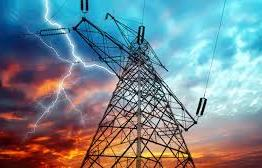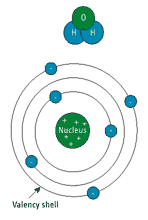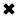# Basic Electricity - Electrical Definition#### Basic Electricity

Basic electricity is described in many ways. When an electric circuit flows through a conductor, a magnetic field (or "flux") develops around the conductor. The highest flux density occurs when the conductor is formed into a coil having many turns. In electronics and electrical technology, a coil is usually known as an inductor. If a steady DC current is run through the coil, you would have an electromagnet - a device with the properties of a conventional magnet, except you can turn it on or off by placing a switch in the circuit.

Basic Electrical Theory
There are four basic electrical quantities that we need to know:

• Current
• Potential Difference (Voltage)
• Power
• Resistance

Electrical Current
Current is a flow of charge. Each electron carries a charge of 1.6 × 10-19 coulombs. This is far too small to be any use, so we consider electricity to flow in packets called coulombs. When there is a flow of 1 coulomb per second, a current of 1 amp is flowing. Current circuit electric is measured in ampères, or amps (A).

Potential Difference
Potential difference is often referred to as voltage. There are several ways of defining voltage; the correct physics definition is energy per unit charge, in other words, how big a job of work each lump of charge can do.

Power in a Circuit
Power in a circuit can be worked out using the simple relationship:

Power (W) = Voltage (V) × Current (A)

Electrical Resistant Behaviour
This is the opposition to the flow of an electric current.

There's reciprocity in the interaction between electron flow and magnetism. If you sweep one pole of a magnet quickly past an electrical conductor (at a right angle to it), a voltage will be momentarily "induced" in the conductor. The polarity of the voltage will depend upon which pole of the magnet you're using, and in which direction it sweeps past the conductor.

This phenomenon becomes more apparent when the conductor is formed into a coil of many turns.Figure 1 shows a coil mounted close to a magnet that is spinning on a shaft. As the north pole of the magnet sweeps past the coil, a voltage is induced in the coil, and, if there is a "complete" circuit, current will flow. As the south pole of the magnet sweeps past, a voltage of opposite polarity is induced, and current flows in the opposite direction.

This is the fundamental operating law of a generator. The output, known as alternating current, is the type of power that electric utility companies supply to businesses and homes. A practical generator would likely have two coils mounted on opposite sides of the spinning magnet and wired together in a series connection. Because the coils are in a series, the voltages combine, and the voltage output of the generator will be twice that of each coil.Figure 2 is a graph of the voltage produced by such a generator as a function of time. Let's assume that this happens to be a 120-volt, 60-Hz generator. The voltage at one point in the cycle momentarily passes through 0 volts, but it's headed for a maximum of 169.7 volts. After that point, the voltage declines, passing through 0 volts, then reverses its polarity, and has a negative "peak" of -169.7 volts.

This curve is known as a sine wave since the voltage at any point is proportional to the sine of the angle of rotation. The magnet is rotating 60 times a second, so the sine wave repeats at the same frequency, making the period of a single cycle one-sixtieth of a second.

Electricity appears in two forms: alternating current (AC) and direct current (DC). Direct current does not change directions-- the electron flow is always from the negative pole to the positive pole -- although as we mentioned before, the electrons themselves don't really "move," it's the holes that are created that "move." Direct current is almost always what is used inside of electronic devices to power the various internal components, but it is a harmful thing in audio signals, which are alternating current. Alternating current does change direction-- standard household electricity is alternating current, because of its flexibility in traveling long distances. It changes direction at a specific frequency-- 60 times per second, or 60 Hz (in the United States, Japan, and a couple of other countries; in Europe the standard is 50 Hz). There is also the issue of the Ohm to consider. Audio signals vary their direction-alternation according to the frequency in question.

AC - ALTERNATING CURRENT
Alternating current or AC electricity is the type of electricity commonly used in homes and businesses throughout the world.

While the flow of electrons through a wire in direct current (DC) electricity is continuous in one direction, the current in AC electricity alternates in direction. The back-and-forth motion occurs between 50 and 60 times per second, depending on the electrical system of the country.

AC is created by an AC electric generator, which determines the frequency. What is special about AC electricity is that the voltage in can be readily changed, thus making it more suitable for long-distance transmission than DC electricity. But also, AC can employ capacitors and inductors in electronic circuitry, allowing for a wide range of applications.

DC - DIRECT CURRENT
In a direct-current system, it's easy to determine voltage because it is nonvarying or varies slowly over time. You can simply make a measurement with a DC voltmeter. But in an AC circuit, the voltage is constantly changing.

Electrical engineers state the voltage of an AC sine wave as the RMS (root-mean-square), a value equal to the peak value of the sine wave divided by the square root of two, which is approximately 1.414. If you know the RMS voltage, you can multiply it by the square root of two to calculate the peak voltage of the curve. If you were to power a light bulb from 120V(RMS) AC, you would get the same amount of light from the bulb as you would by powering it from 120V DC. Yet another device uses electromagnetic induction: the transformer.

Just as an iron core improves the inductance of a coil, it has the same positive effect in a transformer, and most power transformers are wound on iron cores.

In order to understand how electricity is created and works it is necessary to look at how all matter is structured. All matter is made up of molecules that have a certain number of atoms, for example one molecule of water is made up of two atoms of hydrogen and one of oxygen giving a symbol of H 2 O. All other matter also has a symbol like this and is made up of atoms.

To be able to understand electricity however, the atom must be broken down even further into a nucleus, electrons and protons. The nucleus is made up of positively charged protons and neutrally charged neutrons that generally balance the number of negatively charged electrons,which are moving around the nucleus in a similar manner to the planets circling the sun.

The outer ring of electrons is called the Valency Shell and the electrons contained in this ring are called Valence Electrons. These are the electrons which are knocked or forced out to form a flow of electricity. If one or more electrons are moved out of the the atom it will leave the atom with more protons than electrons, which means that the atom will be positively charged.

• One rule that is very prevalent in all forms of electricity, and also magnetism, is that like charges, or poles, repel and unlike charges, poles, will attract. This means that a positively charged object will attract a negatively charged one, but if both charges are the same then they will repel each other.##### Basic Electricity Handbook, Vol. 1• FULLY ILLUSTRATED
• GREAT FOR SCHOOL PROJECTS
• GREAT PRICE: \$5.99
• ...

This 100+ page e-book is a great guide for those who have a basic interest in the field of electricity. This well-illustrated e-book, coupled with some basic knowledge of electricity, will give you a broad theoretical background in this fundamental subject.

CONTENTS
• FUNDAMENTALS OF ELECTRICITY
• HOW DOES ELECTRICITY WORK?
• HOW IS ELECTRICITY GENERATED?
• WHERE DOES ELECTRICITY COME FROM?
• BASIC HOME WIRING
• ELECTRICITY FOR STUDENTS
• ENERGY SAVING TIPS

##### TRAINING EF COURSES

Content Community Connection
Top